# Regular polygons

Martin McBride, 2020-08-14
Tags regular polygon equilateral triangle square pentagon hexagon octagon
Categories maths gcse geometry

A regular polygon is a polygon for which every side is equal and every angle is equal. In this section we will look at some well known regular polygons with 3 to 12 sides. Here is a video:

## 3 sides - equilateral triangle

A three sided shape, of course, is called a triangle. A regular triangle is called an equilateral triangle, and looks like this: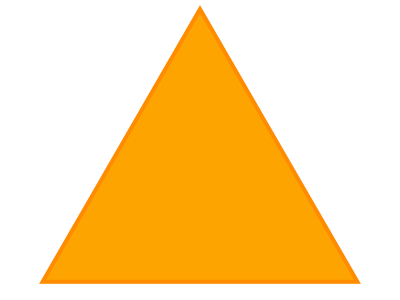## 4 sides - square

A four sided shape is called a quadrilateral. A regular quadrilaterals are more commonly known as squares: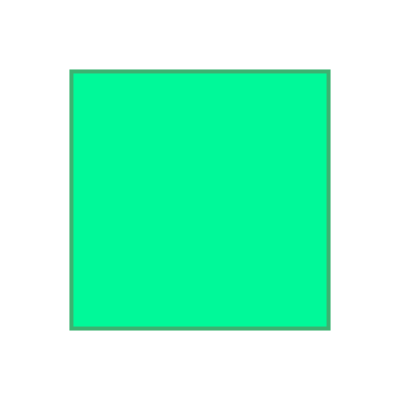## 5 sides - pentagon

A five sided shape is called a pentagon. A regular pentagon looks like this: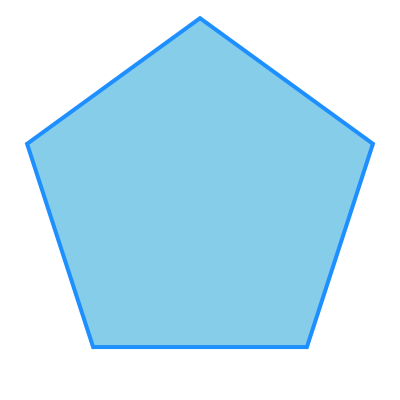## 6 sides - hexagon

A six sided shape is called a hexagon. A regular hexagon looks like this: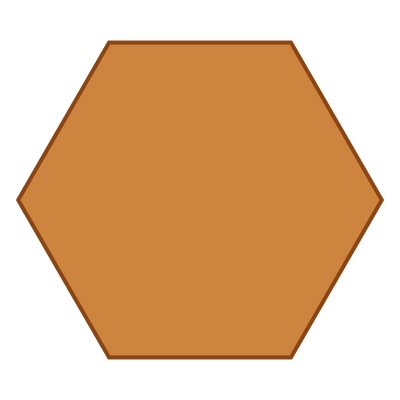## 7 sides - heptagon

A seven sided shape is called a heptagon. A regular heptagon looks like this: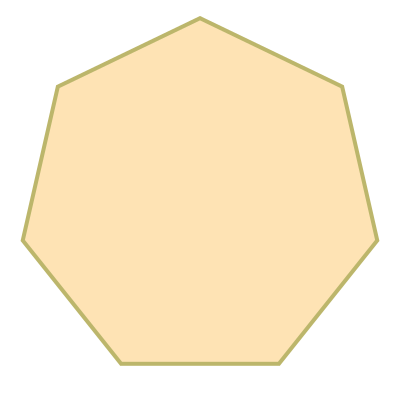## 8 sides - octagon

A eight sided shape is called an octagon. A regular octagon looks like this: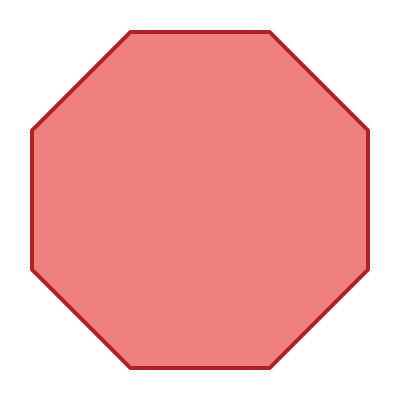## 9 sides - nonagon

A nine sided shape is called a nonagon. A regular nonagon looks like this: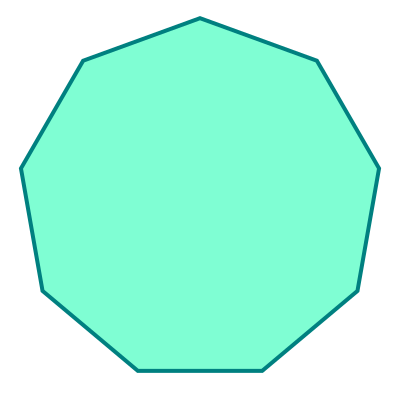## 10 sides - decagon

A ten sided shape is called a decagon. A regular decagon looks like this: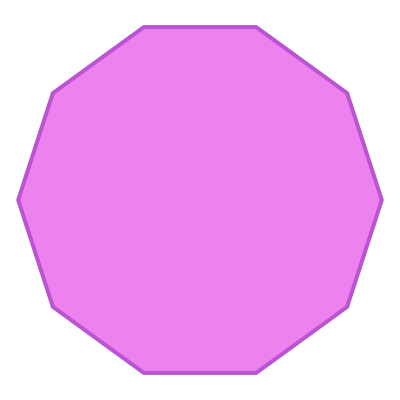## 11 sides - hendecagon

An eleven sided shape is called a hendecagon. A regular hendecagon looks like this: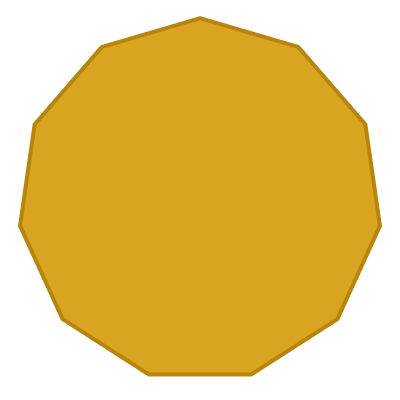## 12 sides - dodecagon

A twelve sided shape is called a dodecagon. A regular dodecagon looks like this: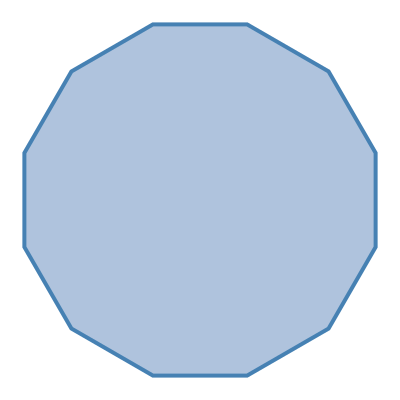## Polygons with more sides

A polygon can have any number of sides, but most do not have well known special names.

## Irregular polygons

An irregular polygon is a polygon where some of the sides and/or angles are different. Some irregular triangles and quadrilaterals have special names (isosceles triangle, rectangle, parallelogram, etc), but irregular shapes with more than four side usually don't.